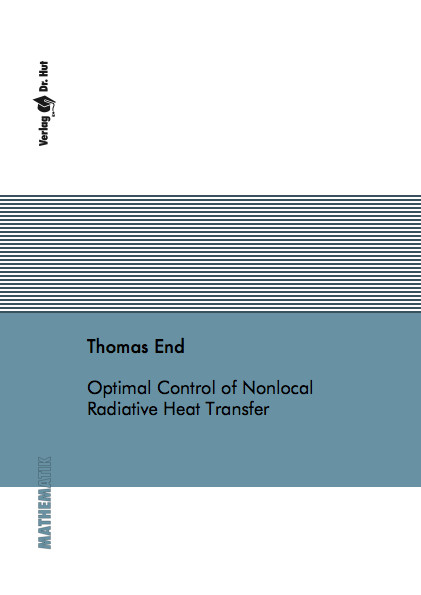Datenbestand vom 25. Mai 2020aktualisiert am 25. Mai 2020

# ISBN 9783843903509Euro 60,00 inkl. 7% MwSt

978-3-8439-0350-9, Reihe Mathematik

Thomas End
Optimal Control of Nonlocal Radiative Heat Transfer

119 Seiten, Dissertation Technische Universität Kaiserslautern (2012), Softcover, A5

## Zusammenfassung / Abstract

This work deals with the coupled radiative heat transfer equations consisting of the radiative transfer equation and the heat transfer equation that model radiative-conductive heat transfer. We analyze a stationary and a quasistationary formulation of the radiative heat transfer equations. We prove the existence and uniqueness of solutions to the system. The existence of solutions is proven with help of Schauder's fixed point theorem for the stationary and the quasistationary case. For large enough thermal conductivities, we prove the uniqueness of solutions to the stationary problem. For the quasistationary problem we use a Gronwall argument to prove the uniqueness of solutions. We introduce two stationary optimal control problems and a quasistationary one. For each of these optimal control problems we derive the optimality system and prove the existence of solutions. Results for optically thick regimes are compared to the Rosseland approximation, which is formally derived using asymptotic analysis and the adjoint approach. The optimal control problems are numerically treated with the BFGS method. Finally, we present numerical results for optically thin and optically thick regimes, varying regularization parameters and perform a grid study that shows the independence of the discretization width.